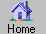Prev Next Index-> contents reference index search external Up-> CppAD CppAD-> Install Introduction AD ADFun preprocessor multi_thread utility ipopt_solve Example speed Appendix Headings-> Syntax Introduction Example Include File Preprocessor Symbols Namespace Contents

$\newcommand{\W}{ \; #1 \; } \newcommand{\R}{ {\rm #1} } \newcommand{\B}{ {\bf #1} } \newcommand{\D}{ \frac{\partial #1}{\partial #2} } \newcommand{\DD}{ \frac{\partial^2 #1}{\partial #2 \partial #3} } \newcommand{\Dpow}{ \frac{\partial^{#1}}{\partial {#2}^{#1}} } \newcommand{\dpow}{ \frac{ {\rm d}^{#1}}{{\rm d}\, {#2}^{#1}} }$,
cppad-20171217: A Package for Differentiation of C++ Algorithms
 One section per web page    All sections in one web page (fast to load)    (slow to load) Math displayed using MathJax    cppad.htm    _printable.htm Math displayed using MathML    cppad.xml    _printable.xml

Syntax  # include <cppad/cppad.hpp>

Introduction
We refer to the step by step conversion from an algorithm that computes function values to an algorithm that computes derivative values as Algorithmic Differentiation (often referred to as Automatic Differentiation.) Given a C++ algorithm that computes function values, CppAD generates an algorithm that computes its derivative values. A brief introduction to Algorithmic Differentiation can be found in wikipedia . The web site autodiff.org is dedicated to research about, and promoting the use of, AD.
1. CppAD uses operator overloading to compute derivatives of algorithms defined in C++. It is distributed by the COIN-OR Foundation with the Eclipse Public License EPL-1.0 or the GNU General Public License GPL-3.0 . Testing and installation is supported for Unix, Microsoft, and Apple operating systems. Extensive user and developer documentation is included.
2. An AD of Base operation sequence is stored as an AD function object which can evaluate function values and derivatives. Arbitrary order forward and reverse mode derivative calculations can be preformed on the operation sequence. Logical comparisons can be included in an operation sequence using AD conditional expressions . Evaluation of user defined unary discrete functions can also be included in the sequence of operations; i.e., functions that depend on the independent variables but which have identically zero derivatives (e.g., a step function).
3. Derivatives of functions that are defined in terms of other derivatives can be computed using multiple levels of AD; see mul_level.cpp for a simple example and mul_level_ode.cpp for a more realistic example. To this end, CppAD can also be used with other AD types; for example see mul_level_adolc_ode.cpp .
5. Includes a set of C++ utilities that are useful for general operator overloaded numerical method. Allows for replacement of the testvector template vector class which is used for extensive testing; for example, you can do your testing with the uBlas template vector class.
6. See whats_new for a list of recent extensions and bug fixes.
You can find out about other algorithmic differentiation tools and about algorithmic differentiation in general at the following web sites: wikipedia , autodiff.org .

Example
The file get_started.cpp contains an example and test of using CppAD to compute the derivative of a polynomial. There are many other examples .

Include File
The following include directive       # include <cppad/cppad.hpp> includes the CppAD package for the rest of the current compilation unit.

Preprocessor Symbols
All the preprocessor symbols used by CppAD begin with eight CppAD or CPPAD_.

Namespace
All of the functions and objects defined by CppAD are in the CppAD namespace; for example, you can access the AD types as       size_t n = 2;      CppAD::vector< CppAD::AD<Base> > x(n) You can abbreviate access to one object or function a using command of the form       using CppAD::AD      CppAD::vector< AD<Base> > x(n) You can abbreviate access to all CppAD objects and functions with a command of the form       using namespace CppAD      vector< AD<Base> > x(n) If you include other namespaces in a similar manner, this can cause naming conflicts.

Contents